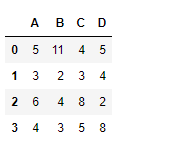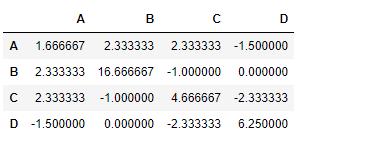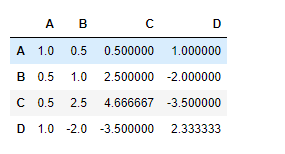Open in App
Not now

# Python | Pandas dataframe.cov()

• Last Updated : 16 Nov, 2018

Python is a great language for doing data analysis, primarily because of the fantastic ecosystem of data-centric python packages. Pandas is one of those packages and makes importing and analyzing data much easier.

Pandas` dataframe.cov()` is used to compute pairwise covariance of columns.
If some of the cells in a column contain `NaN` value, then it is ignored.

Syntax: DataFrame.cov(min_periods=None)

Parameters:
min_periods : Minimum number of observations required per pair of columns to have a valid result.

Returns: y : DataFrame

Example #1: Use `cov()` function to find the covariance between the columns of the dataframe.

Note : Any non-numeric columns will be ignored.

 `# importing pandas as pd``import` `pandas as pd`` ` `# Creating the dataframe``df ``=` `pd.DataFrame({``"A"``:[``5``, ``3``, ``6``, ``4``], ``                   ``"B"``:[``11``, ``2``, ``4``, ``3``],``                   ``"C"``:[``4``, ``3``, ``8``, ``5``],``                   ``"D"``:[``5``, ``4``, ``2``, ``8``]})`` ` `# Print the dataframe``df`

Output :Now find the covariance among the columns of the data frame

 `# To find the covariance ``df.cov()`

Output :Example #2: Use `cov()` function to find the covariance between the columns of the dataframe which are having `NaN` value.

 `# importing pandas as pd``import` `pandas as pd`` ` `# Creating the dataframe``df ``=` `pd.DataFrame({``"A"``:[``5``, ``3``, ``None``, ``4``],``                   ``"B"``:[``None``, ``2``, ``4``, ``3``],``                   ``"C"``:[``4``, ``3``, ``8``, ``5``], ``                   ``"D"``:[``5``, ``4``, ``2``, ``None``]})`` ` `# To find the covariance ``df.cov()`

Output :My Personal Notes arrow_drop_up